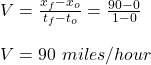## A car travels in a straight line covering a total distance of 90.0 miles in 60.0 minutes. Which one of the following statements concerning t

Question

A car travels in a straight line covering a total distance of 90.0 miles in 60.0 minutes. Which one of the following statements concerning this situation is necessarily true?
A) The velocity of the car is constant.
B) The acceleration of the car must be non-zero.
C) The first 45 miles must have been covered in 30.0 minutes.
D) The speed of the car must be 90.0 miles per hour throughout the entire trip.
E) The average velocity of the car is 90.0 miles per hour in the direction of motion.

in progress 0
2 months 2021-07-27T17:14:33+00:00 1 Answers 32 views 0

E) The average velocity of the car is 90.0 miles per hour in the direction of motion.

Explanation:

Given;

distance covered by the car, d = 90 miles

time taken, t = 60 minutes = 1 hour

The average velocity of the car is given by change in displacement per change in time;

V = Δd / ΔtTherefore, the average velocity of the car is 90.0 miles per hour in the direction of motion.